# Java 17的计算速度比Java 8慢? - marian

22-02-11 banq

Java中的计算任务
Java 的性能不如 Python，但更方便用于计算。让我们进行最简单的实验，看看现代 Java 的计算能力发生了什么变化。

 ```public class Main { static long fibonacci(long n) {     if (n <= 1) {       return n;     } else {       return fibonacci(n - 1) + fibonacci(n - 2);     }   } class Fibonacci extends RecursiveTask { final long n; Fibonacci(long n) {       this.n = n;     } protected Long compute() {       if (n <= 10) {         return fibonacci(n);       }       Fibonacci f1 = new Fibonacci(n - 1);       f1.fork();       Fibonacci f2 = new Fibonacci(n - 2);       return f2.compute() + f1.join();     }   } Map> results = new HashMap<>(); void execute(IntFunction code, int num, String name) {     long start = System.currentTimeMillis();     long r = code.apply(num);     long time = System.currentTimeMillis() - start;     results.computeIfAbsent(name, k -> new LinkedList<>()).add(time);     System.out.println(r + " in " + time + " ms");   } void run(int num, int repeats) {     for (int i = 0; i < repeats; i++) {       execute(n -> fibonacci(n), num, "one");       execute(n -> new Fibonacci(n).compute(), num, "multi");     }       results.forEach((k, v) ->     System.out.println(k + " " + v.stream().mapToLong(l -> l).average().getAsDouble()));   } public static void main(String... args) {     new Main().run(Integer.valueOf(args),  Integer.valueOf(args));   } } ```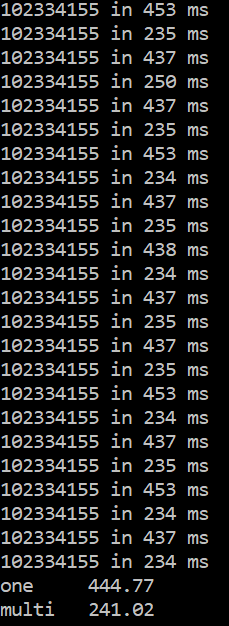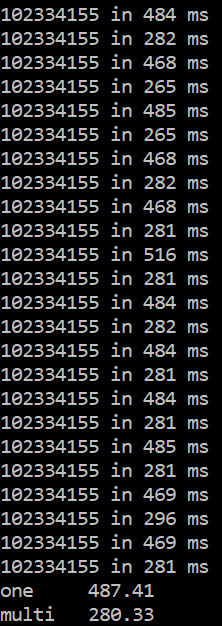Java 17 在计算任务上似乎慢 (487–444)/444=9% 和 (280–241)/241=16%。这难以置信。让我们尝试另一个数字，45，但只计算了 20 次，因为如您所见，计算需要时间。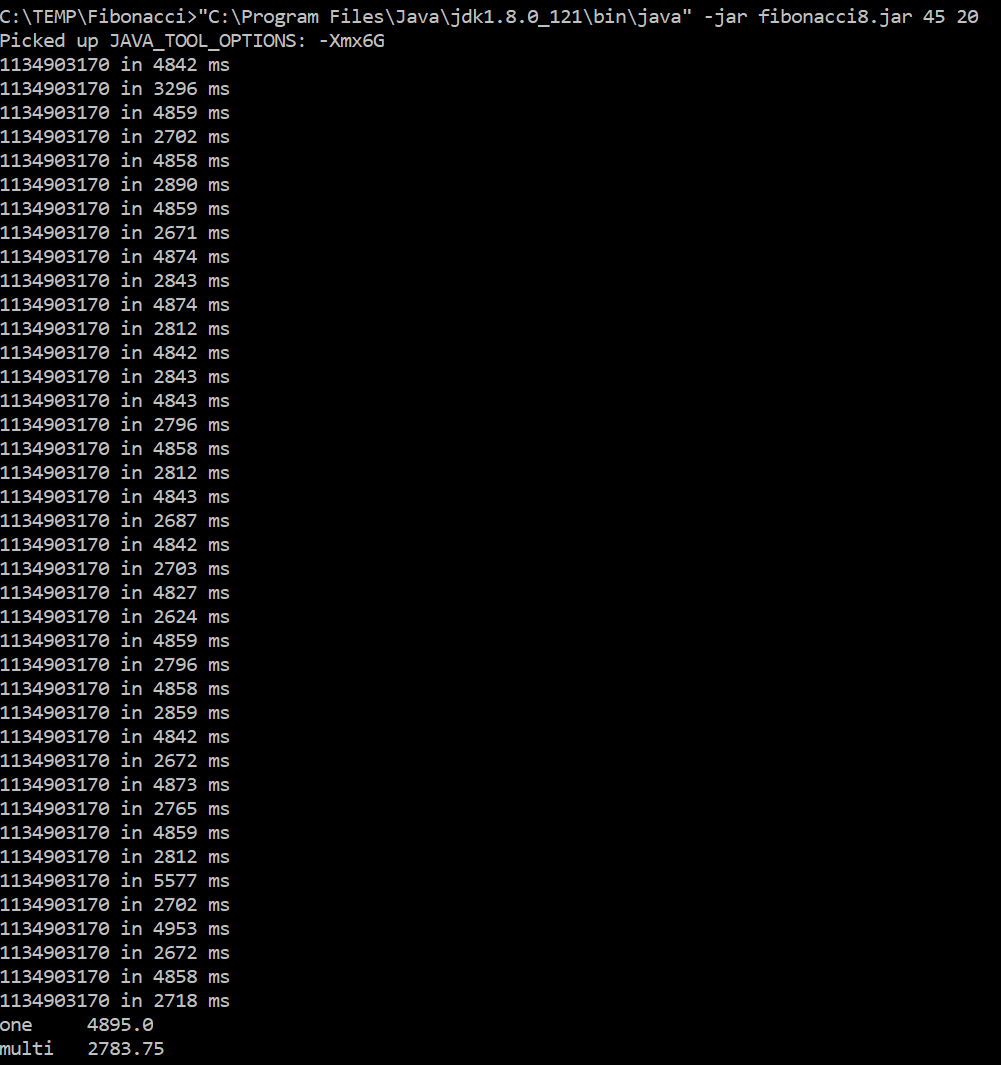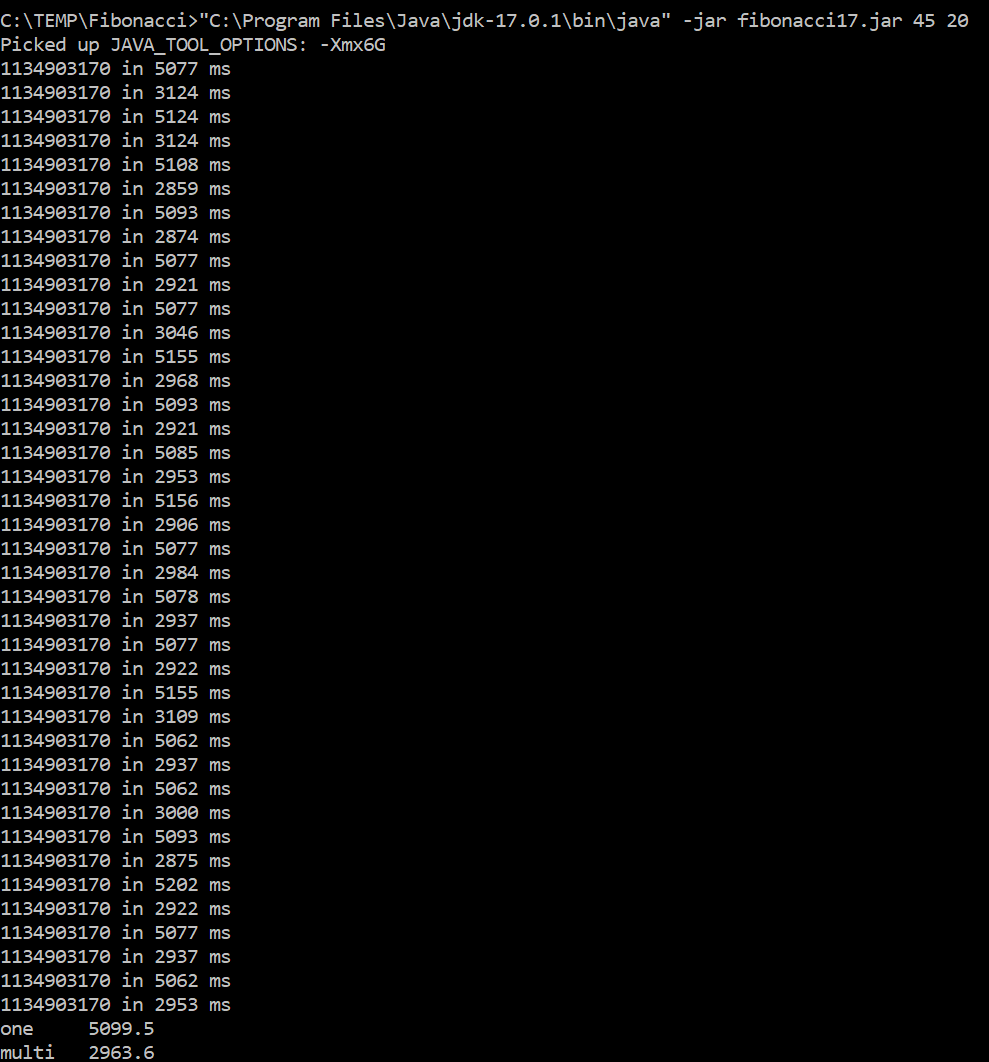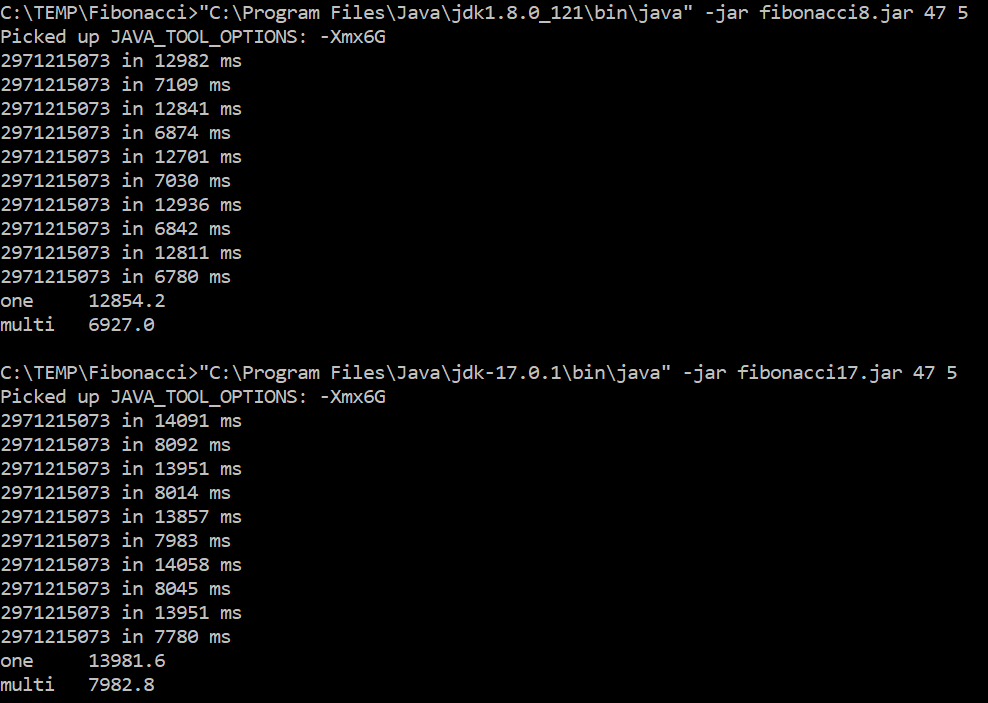1